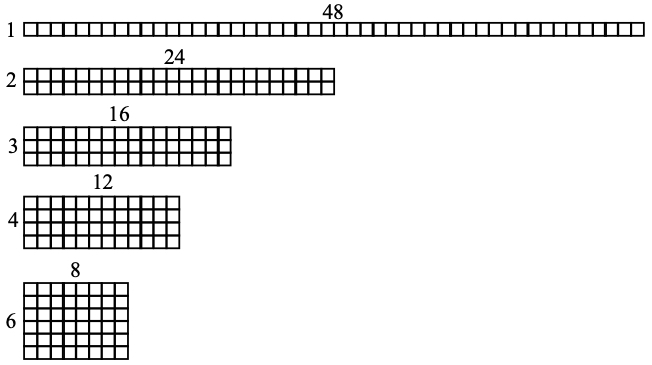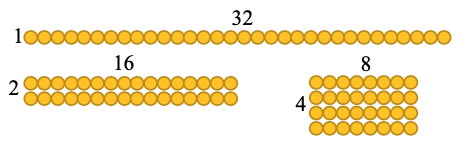# MA.4.AR.3.1Export Print
Determine factor pairs for a whole number from 0 to 144. Determine whether a whole number from 0 to 144 is prime, composite or neither.

### Clarifications

Clarification 1: Instruction includes the connection to the relationship between multiplication and division and patterns with divisibility rules.

Clarification 2: The numbers 0 and 1 are neither prime nor composite.

General Information
Subject Area: Mathematics (B.E.S.T.)
Strand: Algebraic Reasoning
Status: State Board Approved

## Benchmark Instructional Guide

### Terms from the K-12 Glossary

• Composite Number
• Factors
• Prime Number

### Vertical Alignment

Previous Benchmarks

Next Benchmarks

### Purpose and Instructional Strategies

The purpose of this benchmark is for students to begin understanding factors of whole numbers which sets the foundation for determining prime factorization in grade 6 (MA.6.NSO.3.4).
• This benchmark also refers to prime and composite numbers. Prime numbers have exactly two factors, the number one and the number itself (e.g., the number 13 has the factors of 1 and 13 only so it is a prime number). Composite numbers have more than two factors.
• For example, 14 has the factors of 1, 2, 7 and 14. Since this number has more factors than 1 and 14, it is a composite number.
• Instruction may allow students to use divisibility rules to determine the factors of a number.
• All numbers are divisible by 1.
• All even numbers are divisible by 2.
• A number is divisible by 3 if the sum of the digits is divisible by 3.
• A number is divisible by 4 if the 2-digit number in the tens and ones places is divisible by 4.
• A number is divisible by 5 if the number in the ones place is a 0 or 5.
• A number is divisible by 6 if it is an even number and the sum of the digits is divisible by 3.
• A number is divisible by 9 if the sum of the digits is divisible by 9.
• Students should use models (arrays) to determine why a number would be prime or composite.

### Common Misconceptions or Errors

• Students may think of the number 1 as a prime number. It is neither prime nor composite.
• Students may also think that all odd numbers are prime.
• Some students may think that larger numbers have more factors. Have students share all factor pairs and how they found the factors.

### Strategies to Support Tiered Instruction

• Instruction includes opportunities to identify the factor pairs of whole numbers from 0- 144 with teacher guidance. Students also determine if the number is prime, composite or neither. The teacher refers to the divisibility rules shown above in “Purpose and Instructional Strategies” to support students as they determine the factors of the given number. Students use manipulatives and models (e.g., counters and arrays) to identify factors of the given number.
• For example, the teacher displays the following number: 48. Students draw arrays to determine the factors of 48 (1, 2, 3, 4, 6, 8, 12, 16, 24, 48). Students may use multiplication and skip counting to check factor pairs (e.g., 5 can be ruled out as a factor because 5 × 9 = 45 and 5 × 10 = 50). The teacher asks students if 48 is prime, composite, or neither (composite). This is repeated with additional whole numbers from 0-144.• For example, the teacher displays the following number: 32. Students are provided 32 counters and use them to create arrays to determine the factors of 32 (1, 2, 4, 8, 16, 32). Students may use multiplication and skip counting to check factor pairs (e.g., 5 can be ruled out as a factor because 5 × 6 = 30 and 5 × 7 = 35). Students may explore using counters to rule out additional numbers as factors (e.g., if you make 6 rows with a total of 32 counters, there will not be an even number in each row). The teacher asks students if 32 is prime, composite, or neither (composite). This is repeated with additional whole numbers from 0- 144.Find all the factors for the numbers between 20 and 30. What is the greatest prime number in this set of primes? What is the least prime number in this set of primes?

### Instructional Items

Instructional Item 1

Select all the statements are true about the number 84.
• a. 42 is a factor of 84.
• b. 84 is a composite number.
• c. 84 has exactly 4 distinct factor pairs.
• d. The prime factors of 84 are 2, 6 and 7.
• e. 84 can be written as the product 2 × 2 × 3 × 7.

*The strategies, tasks and items included in the B1G-M are examples and should not be considered comprehensive.

## Related Courses

This benchmark is part of these courses.
5012060: Mathematics - Grade Four (Specifically in versions: 2014 - 2015, 2015 - 2022, 2022 and beyond (current))
7712050: Access Mathematics Grade 4 (Specifically in versions: 2014 - 2015, 2015 - 2018, 2018 - 2022, 2022 and beyond (current))
5012055: Grade 3 Accelerated Mathematics (Specifically in versions: 2019 - 2022, 2022 and beyond (current))
5012015: Foundational Skills in Mathematics 3-5 (Specifically in versions: 2019 - 2022, 2022 and beyond (current))

## Related Access Points

Alternate version of this benchmark for students with significant cognitive disabilities.
MA.4.AR.3.AP.1: Explore factor pairs for a whole number. Factors may not exceed single-digit whole numbers.

## Related Resources

Vetted resources educators can use to teach the concepts and skills in this benchmark.

## Educational Games

Arithmetic Workout:

This tutorial will help you to brush up on your multiplication, division and factoring skills with this exciting game.

Type: Educational Game

Sigma Prime: A Prime Factorization Game:

This fun and engaging game will test your knowledge of whole numbers as prime or composite.  As you shoot the asteroids with a particular factor, the asteroids will break down by that chosen factor.  Keep shooting the correct factors to totally eliminate the asteroids.  But be careful, shooting the wrong factor has consequences!

Type: Educational Game

## Formative Assessments

Find All the Factor Pairs:

Students are asked to find all the factor pairs for each of four given numbers.

Type: Formative Assessment

Factor Pairs:

Students are asked to find all the factor pairs of a given number and identify the number as a multiple of the factors.

Type: Formative Assessment

Prime or Composite:

Students are asked to determine whether each of four given numbers is prime or composite and justify their choices.

Type: Formative Assessment

## Lesson Plans

Fantastic Factors:

In this lesson, students will start out using manipulatives to create arrays which will be used to find factor pairs. Students will use a T-Chart to determine the factor pairs of numbers. Students will then use their T-Charts to determine if a number is prime or composite.

Type: Lesson Plan

Hooray for arrays!:

Students will identify prime and composite numbers through arrays. The lesson begins with a fun situational story.

Type: Lesson Plan

Using Rectangles to Find Prime and Composite Numbers:

In this lesson, students will be using rectangles to find prime and composite numbers.  Students will draw different rectangles for the area of a given set of numbers.  They will determine the factor pairs for each number in the given set and use them to discover the meaning of prime and composite numbers.

Type: Lesson Plan

Numbers Grow Here:

The students will use prior knowledge of the area formula to design a garden with a  area. Students will compare gardens and note that rectangles with the same area could have different dimensions.

Type: Lesson Plan

Fun with Factors:

This is an introductory lesson addressing factors for number 1-20. Factoring will lead students to discover differences and similarities between prime and composite numbers. Students will also discuss five claims about factors, prime, and composite numbers and create support from examples and non-examples to determine whether these claims are valid or invalid.

Type: Lesson Plan

Searching for the Primes:

Students will use a 100s chart to sort out the prime numbers under 100 using the Sieve of Eratosthenes method.

Type: Lesson Plan

Prime Factorization - From Fingerprints to Factorprints:

This activity provides an introduction to composite numbers and prime numbers through factorization.

Type: Lesson Plan

Area and Perimeter of Rectangles Investigations:

Students will determine the validity of the statement, "All rectangles with the same area will have the same perimeter" through two investigations.

Type: Lesson Plan

## Original Student Tutorial

Prime and Composite Backyard Dilemma :

Discover what makes prime and composite numbers unique thanks to an interesting backyard problem in this interactive tutorial.

Type: Original Student Tutorial

Identifying Multiples:

The goal of this task is to work on finding multiples of some whole numbers on a multiplication grid. After shading in the multiples of 2, 3, and 4 on the table, students will see a key difference.  The focus can be on identifying patterns or this can be an introduction or review of prime and composite numbers.

## Tutorial

Recognizing Prime and Composite Numbers:

This Khan Academy tutorial video reviews how to determine if a number is prime or composite.

Type: Tutorial

## MFAS Formative Assessments

Factor Pairs:

Students are asked to find all the factor pairs of a given number and identify the number as a multiple of the factors.

Find All the Factor Pairs:

Students are asked to find all the factor pairs for each of four given numbers.

Prime or Composite:

Students are asked to determine whether each of four given numbers is prime or composite and justify their choices.

## Original Student Tutorials Mathematics - Grades K-5

Prime and Composite Backyard Dilemma :

Discover what makes prime and composite numbers unique thanks to an interesting backyard problem in this interactive tutorial.

## Student Resources

Vetted resources students can use to learn the concepts and skills in this benchmark.

## Original Student Tutorial

Prime and Composite Backyard Dilemma :

Discover what makes prime and composite numbers unique thanks to an interesting backyard problem in this interactive tutorial.

Type: Original Student Tutorial

## Educational Games

Arithmetic Workout:

This tutorial will help you to brush up on your multiplication, division and factoring skills with this exciting game.

Type: Educational Game

Sigma Prime: A Prime Factorization Game:

This fun and engaging game will test your knowledge of whole numbers as prime or composite.  As you shoot the asteroids with a particular factor, the asteroids will break down by that chosen factor.  Keep shooting the correct factors to totally eliminate the asteroids.  But be careful, shooting the wrong factor has consequences!

Type: Educational Game

Identifying Multiples:

The goal of this task is to work on finding multiples of some whole numbers on a multiplication grid. After shading in the multiples of 2, 3, and 4 on the table, students will see a key difference.  The focus can be on identifying patterns or this can be an introduction or review of prime and composite numbers.

## Tutorial

Recognizing Prime and Composite Numbers:

This Khan Academy tutorial video reviews how to determine if a number is prime or composite.

Type: Tutorial

## Parent Resources

Vetted resources caregivers can use to help students learn the concepts and skills in this benchmark.